Next: About this document ... Up: lab_template Previous: lab_template

Subsections

# Parametric curves in the plane

## Purpose

The purpose of this lab is to give you practice with parametric curves in the plane and in visualizing parametric curves as representing motion.

## Background

A parametric curve in the plane is defined as an ordered pair,
, of functions, with
representing the
coordinate and
the
coordinate. Parametric curves arise naturally as the solutions of differential equations and often represent the motion of a particle or a mechanical system. They also often arise in studying oscillations in electrical circuits.

For example, neglecting air resistance, the position of a projectile fired from the origin at an initial speed of
and angle of inclination
is given by the parametric equationswhereis time and
is the acceleration due to gravity.

To help you to visualize parametric curves as representing motion, a Maple routine called ParamPlot has been written. It uses the Maple animate command to actually show the particle moving along its trajectory. You actually used this command before for the lab on polar coordinates. Examples are in the Getting Started worksheet.

### Derivatives and the slope

The graph of a parametric curve may not have a slope at every point on the curve. When the slope exists, it must be given by the formula from class.It is clear that this formula doesn't make sense if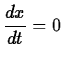at some particular value of. If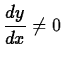at that same value of, then it turns out the graph has a vertical tangent at that point. If bothand
are zero at some value of, then the curve often doesn't have a tangent line at that point. What you see instead is a sharp corner, called a cusp.An example of this appears in the first exercise.

### Arc length

As mentioned above, parametric curves often represent the motion of a particle or mechanical system. As we will see in class, when we think of a parametric curve as representing motion, we need a way to measure the distance traveled by the particle. This distance is given by the arc length,
, of a curve. For a parametric curve
,, the arc length of the curve for
is given below.
While the concept of arc length is very useful for the theory of parametric curves, it turns out to be very difficult to compute in all but the simplest cases.

## Maple Commands

There are a variety of ways to work with parametric equations in Maple. There is an animation command that shows how the graph is plotted over t. For example the parabola
can be written parametrically in different ways two of them areand
>with(plots):
>with(CalcP7):
>implicitplot(x^2=y,x=-2..2,y=0..4,scaling=constrained);
>ParamPlot([t,t^2],t=-2..2,scaling=constrained);
>ParamPlot([-t,t^2],t=-2..2,scaling=constrained);

The ParamPlot command produces an animated plot. To see the animation, execute the command and then click on the plot region below to make the controls appear in the Context Bar just above the worksheet window. To enter a function parametrically
>f:=t->[t*cos(3*t),t^2];
>VPlot(f(t),t=-2*Pi..2*Pi);


## Exercises

1. The cycloid is a famous example of a parametric curve having several important applications. Use the ParamPlot command to animate the cycloid
,
over the interval
. The sharp points in the graph at
,
, andare called cusps. Use the formula for the slope of a parametric curve to explain why it makes sense for the cusps to occur only at these values of. That is, verify that the curve has a slope at all other values ofin the interval.

2. The family of parametric curveswhere
and
are positive integers, is an example of what is called a Lissajous figure. Use ParamPlot to plot the three cases
,and
and describe what you see.

3. The parametric description
,
,is the ellipseFirst show that the two are the same shape by plotting them with the commands implicitplot and VPlot. Use the formula above to set up an integral for the arc length of the ellipse. You should find that Maple can't do the integral exactly. This isn't because Maple is stupid, but because this integral really can't be done analytically. You can get a numerical approximation to the integral by putting an evalf command on the outside of the int command.Next: About this document ... Up: lab_template Previous: lab_template
Jane E Bouchard
2006-11-30